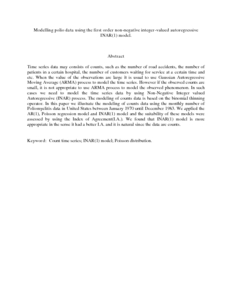# Modelling polio data using the first order non-negative integer-valued autoregressive INAR(1) model.

## Citation

Vazifedan, Turaj and Shitan, Mahendran (2012) Modelling polio data using the first order non-negative integer-valued autoregressive INAR(1) model. International Journal of Modern Physics: Conference Series, 9 (-). pp. 232-239. ISSN 2010-1945

## Abstract

Time series data may consists of counts, such as the number of road accidents, the number of patients in a certain hospital, the number of customers waiting for service at a certain time and etc. When the value of the observations are large it is usual to use Gaussian Autoregressive Moving Average (ARMA) process to model the time series. However if the observed counts are small, it is not appropriate to use ARMA process to model the observed phenomenon. In such cases we need to model the time series data by using Non-Negative Integer valued Autoregressive (INAR) process. The modeling of counts data is based on the binomial thinning operator. In this paper we illustrate the modeling of counts data using the monthly number of Poliomyelitis data in United States between January 1970 until December 1983. We applied the AR(1), Poisson regression model and INAR(1) model and the suitability of these models were assessed by using the Index of Agreement(I.A.). We found that INAR(1) model is more appropriate in the sense it had a better I.A. and it is natural since the data are counts.Preview
PDF (Abstract)
Modelling polio data using the first order nonnegative integer-valued autoregressive INAR(1) model..pdfView Item